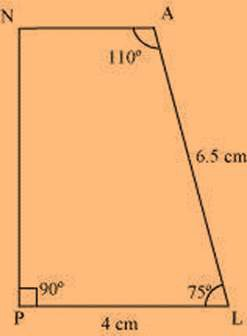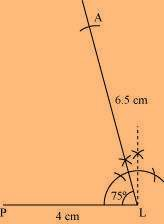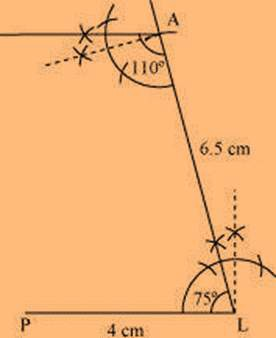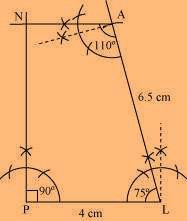# Construct the following quadrilateral: Quadrilateral PLAN PL = 4 cm LA = 6.5 cm ∠P = 90° ∠A = 110° ∠N = 85°

Solution

The sum of the angles of a quadrilateral is 360°.

In quadrilateral PLAN, ∠P + ∠L + ∠A + ∠N = 360°

90° + ∠L + 110° + 85° = 360°

285° + ∠L = 360°

∠L = 360° − 285° = 75°

Rough sketch of the quadrilateral PLAN:We can consct the quadrilateral using the given steps

(1) Draw a line segment PL of 4 cm and draw an angle of 75º at point L. As vertex A is 6.5 cm away from vertex L, cut a line segment LA of 6.5 cm from this ray.(2) Again draw an angle of 110º at point A.(3) Draw an angle of 90º at point P. This ray will meet the previously drawn ray from A at point N.(0)(0)